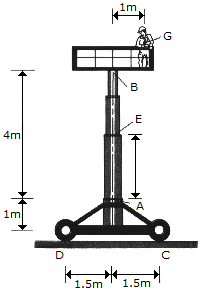# Engineering Mechanics - Internal Forces - Discussion

### Discussion :: Internal Forces - General Questions (Q.No.2)

2.The work platform supports an 80-kg man having a mass center at G. Determine the axial force, shear force, and bending moment at point E of the telescopic column AB due to the load of the man only.

 [A]. AE = 785 N C, VE = 0, ME = 785 N-m [B]. AE = 785 N C, VE = 392 N, ME = 0 [C]. AE = 785 N T, VE = 392 N, ME = 0 [D]. AE = 785 N T, VE = 0, ME = 785 N-m

Explanation:

No answer description available for this question.

 Sheel said: (Aug 25, 2014) Moment about E = 80*9.81*1. = 785Nm. Axial force = 80*9.81N. No shear force.

 Kartik said: (Sep 2, 2017) Yes, the force is eccentric but we can replace it by the axial force and moment.